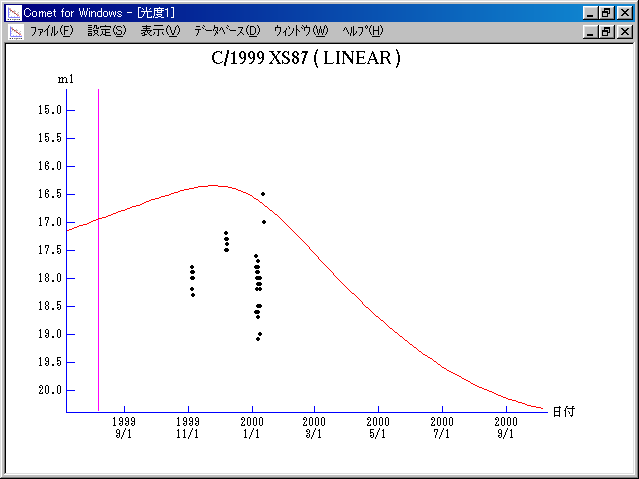# \$B%j%K%"WB@1(B

C/1999 XS87 ( LINEAR )###\$B%W%m%U%#!<%k(B

 \$BH/8+F|(B 1999\$BG/(B12\$B7n(B7\$BF|(B \$BH/8+8wEY(B 17.3\$BEy(B \$BH/8+ Lincoln Laboratory Near-Earth Asteroid Research project

###\$B###\$B50F;MWAG(B

```   The following improved orbital elements by Kenji Muraoka, are
from 58 observations 1999 Nov. 4 to 2000 Feb. 5, perturbations
by 9 Planets, Moon and 5 minor planets were taken into account.
The mean residual is +/- 0.60 arc seconds.

Epoch  =  1999 Aug. 10.0  TT       JDT = 2451400.5
T  =  1999 Aug.  6.57420       +/- 0.01000 (m.e.) TT
Peri. =  151.33550                +/- 0.00471
Node  =  266.73006                +/- 0.00578   (2000.0)
Incl. =   14.84286                +/- 0.00191
q  =    2.7714038              +/- 0.0015246 AU
e  =    0.8412226              +/- 0.0000633
a  =   17.4546528              +/- 0.0066162 AU
n  =    0.01351566             +/- 0.00000768
P  =   72.923                  +/- 0.0414625  years
(+/-15.14 days)
```

###\$B8wEYJQ2=(B

```        m1 = 10.0 + 5 log\$B&\$(B + 10.0 log r
```##### \$B50F;MWAG\$OB<2,7r<#;a\$N7W;;\$K\$h\$k\$b\$N\$G\$9!#(B \$B@1?^\$O(B StellaNavigator Ver.2.0 for Windows (\$B%"%9%H%m%"!<%D(B \$BJTCx(B / \$B%"%9%-!<=PHG6I4)(B) \$B\$G:n@.\$7\$?\$b\$N\$G\$9!#(B \$B8wEY%0%i%U\$O(BComet for Windows\$B\$G:n@.\$7\$?\$b\$N\$G\$9!#(B